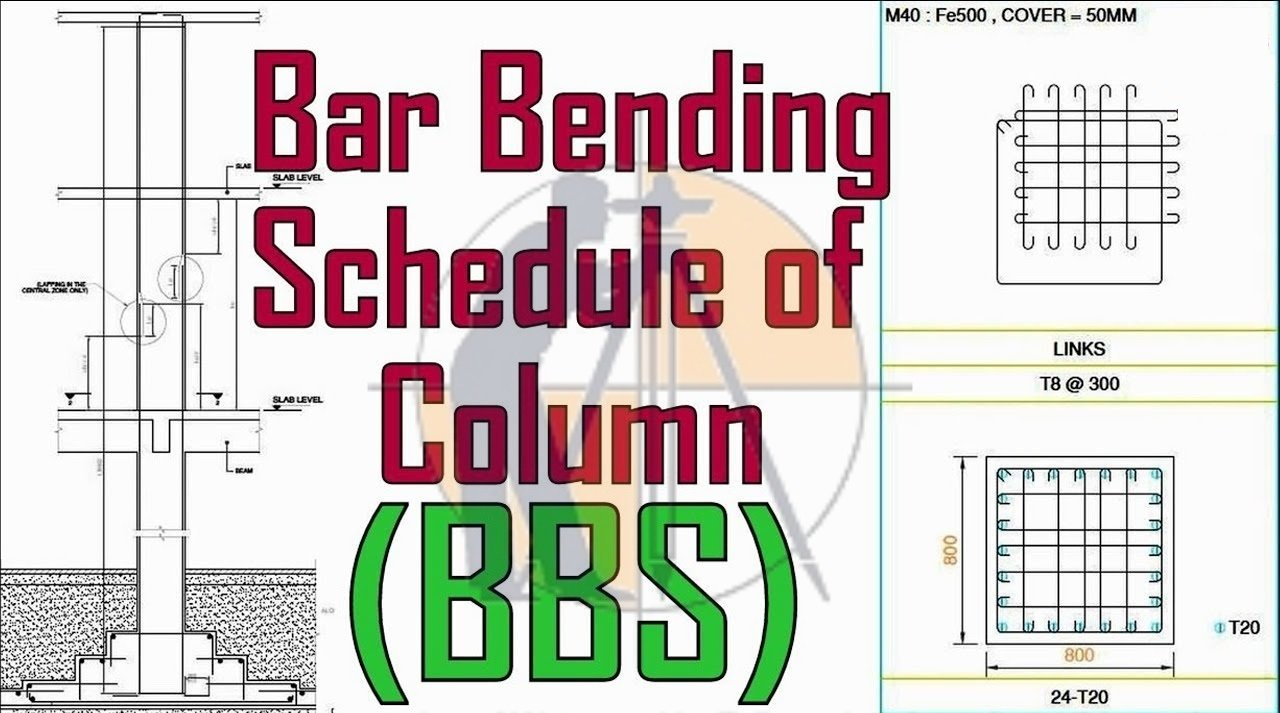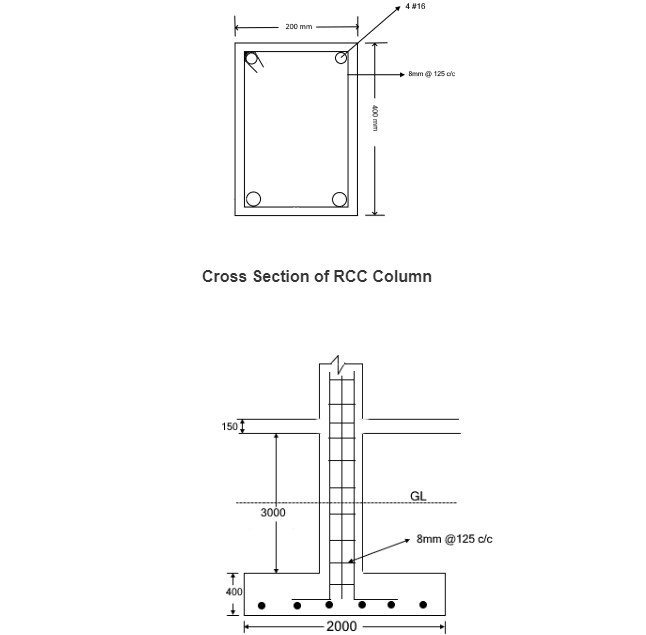Breaking News

# BBS for RCC Column

## Bar Bending Schedule for R.C.C Column

To preparing B.B.S for a column. there are some criteria and assumptions that require to be met Let’s get started.### Forces on Column

U should know the normal forces action on a column.

Just look at the diagram that is given below:

If you see the shear force and bending moment diagram, the column has less moments at center & more at bottom and top.

When bending moment is maximum not at any time tie a column at top and bottom every rime tie the column at center.

At site tie the bars at the height of 4 to 5 feet. The height of roof should not be more than 11 feet.

• Use less cutting of steel and provide desirable lapping.
• Lap another steels at same time.
• Overlap the top steels with bottom steels.
• Between the laps end to end spacing should be provided.

### Bar Bending Schedule (B.B.S) used by

B.B.S is used by different kind of peoples and officials on construction site which is given below:

• Quantity surveyor
• Steel fixer
• Contractor
• Project manager
• Inspectors
• Clerks of construction

In construction site all Estimation & Costing operation is the responsibilities of Quantity Surveyor. The B.B.S gives the details to Quantity Surveyor for finding out the number of steel its type, size and shape. Contractor are using (B.B.S) for ordering the steel bar for proceeding the construction.

2. ### How to Calculate Staircase Concrete & Bar Bending Schedule And Staircase Reinforcement Details

Steel fixer using  (B.B.S) for doing their work or installing the bar in column, beam or slab according to the (B.B.S) that how much cut length or bending of bar will be needed and which size of steel should be use. The inspector and clerks of construction refers to the B.B.S to make are that the reinforcement work in the field is in proper with the design reinforcement as per drawing.

## Bar Bending Schedule for Column### Details from the diagram

• Thickness of slab is150 mm
• Floor height is 3 m or 3000 mm
• Footing depth is 400 mm
• Development Length is 50d
• Column has 4 numbers of 16 mm dia bars
• Stirrup spacing is 8mm @ 150 mm C/C
• Concrete Cover of Footing is 40 mm
• Concrete Cover of slab is 20 mm

### Steps for B.B.S of Column

Generally, there are five steps for preparing the B.B.S of column these five is given below:

• Find the length of Vertical Bar
• Find out lapping
• Cutting Length of Stirrups
• Number of Stirrups
• B.B.S

### Find the length of  Vertical Bar

Length of Vertical bar = Development length (Ld) + footing depth + Floor Heights + Slab Thicknesses + Overlap Length

= (12×16) +400+ (3000) +(150) +(50×16)

= 4734 mm or 4.73 m

1. Calculation Method For Find Out The Overlapping Length Of Beam, Column And Slab

### Now We Will find the length of the One Vertical bar

The total length of the vertical bar = 4732 x number of steels in column

The total length of the vertical bar = 4732 x 4

The total length of the vertical bar = 18928 mm

##### Find out lapping:

length of lapping is 50d

Lapping length = 50 x Dia of steel

Length of lapping = 50×16 = 1000 mm.

We know that the length of typical steel is 12.25 m or 40 feet length

Total Length of Vertical Bar = 18.9 m that is more than 12.25 m or 40 feet so lapping should be provided.

Length of lapping is 1000 mm the result will be 18.928 + 1 = 19.928 m

It is mentioned already in this article, consider the points while lapping the bar. There is no one right process we can use. Just remember the lines.

1. Don’t tie a column at bottom and also at top
2. Lap at another bars every time
3. Overlap top bars with bottom bars always

### Cutting Length of Stirrups

We already discussed cut length of stirrup in B.B.S for beam article.

Length of One Hook = 9d

Cutting length of Stirrup = (Perimeter of stirrup – concrete cover) + Number of Hooks

Cutting length of stirrup = 2(a- cc) + 2(b-cc) + 2 hooks

Cutting length of stirrup = 2(500- 25) + 2 (200-25) +(2x9d)

= (2×475) +(2×175) + (2×9×16)

Cutting length of Stirrup = 1588 mm

### Number of Stirrups:

Number of stirrups = (Total length of Column ÷ spacing of stirrups) +1

Number of stirrups = 4773 ÷ 125 + 1

Number of stirrups = 39 numbers

### B.B.S=

The B.B.S of column is shown below in table

 S. NO Bar Mark Bar dia No of bars Length (m) unit weight(m/kg) weight of steel (Kg) 1 Vertical Bar 16 4 4.773 1.57 29.97 2 Stirrups Bar 8 39 1.588 0.395 24.46 Total Weight of Reinforced Concrete Column 54.43

## Land Surveying & Architects

### THANKS.